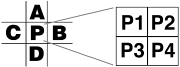After developing the Scale2x algorithm in the 2001 for the AdvanceMAME project, some years later, I found in the Wikipedia article Pixel art scaling algorithms that an already developed algorithm called EPX was producing the exact same result of Scale2x!

Really a surprise, as in fact, they are producing the same graphic effect despite the Scale2x algorithm definition is considerably different and faster!

The following is an extract of the MacTech article Fast Blit Strategies: A Mac Programmer's Guide, vol15, issue6, 1999 describing the EPX algorithm developed by Eric Johnston in the 1992 when working for LucasArts.

Another common speed-multiplying technique is pixel doubling, which is where each pixel of the source image (whether it's a sprite, icon, underlay image, or whatever) is drawn as a 2x2 tile onscreen. Essentially, you're scaling a quarter-size image up to full size - hence, the potential exists for a 4:1 speed boost. The downside to this technique is that it gives a "chunky pixel" look that can be annoying; but there happens to be a useful workaround, in the form of the 'epx' antialiasing technique pioneered by LucasArts' Eric Johnston. Figure 1 shows how it works.The problem is this: how to scale pixel 'P' to be four times its original size, but without all four new pixels necessarily being the same color. (We want some antialiasing.) In Figure 1, the tic-tac-toe grid on the left represents the pixel 'P' and its north, south, east, and west neighbors in the source (offscreen buffer) image. The 2x2 tile on the right represents the new (onscreen) pixels, which will derive from P. The question is how to color P1, P2, P3, and P4 without giving the "chunky pixel" look.

The answer is to base P1 on the back-buffer pixels 'A' and 'C'; base P2 on 'A' and 'B'; base P3 on 'C' and 'D'; and base P4 on 'D' and 'B'. We say that 'A' and 'C' are the "parents" of P1, 'D' and 'B' are the parents of P4, etc., and likewise, P1 is the child of 'A' and 'C', and so forth. The rule to follow is this: If any two parents are equal in color, then make the child that color. Otherwise, let the child be the color of 'P'. Also, if at least three of the parents (A, B, C, D) are identical, do nothing: just let P1 = P2 = P3 = P4 = P. (Johnston discusses this technique on page 692 of Tricks of the Mac Game Programming Gurus, Hayden Books, 1995.)